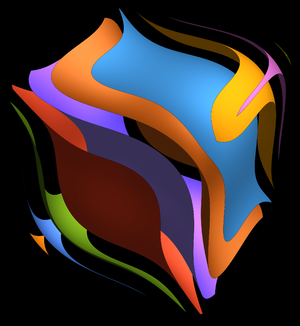# x3 + y3 + z3 = 42

 We know already since Fermat that x3 + y3 + z3 =0 has no solution with x y z different from zero. What about small numbers like x3 + y3 + z3=12 ? Here we can just search in cubes like ```Do[If[x^3+y^3+z^3==12,Print[{x,y,z}]], {x,-15,15},{y,x,15},{z,y,15}] ``` and get the solution x=-11,y=7,z=10. But finding the solution is not always that easy. The register mentions a solution to the sum of three cubes problems for k=42. See also the Bristol press announcement from today. The challenge was to give for every number k between 1 and 100 the solution of x3 + y3 + z3 = k. The last one was k=42. No wonder, the answer to the ultimate question is difficult to find. Andrew Booker from the University of Bristol and Andrew Sutherland from MIT have now found it:```x=-80538738812075974;y=80435758145817515;z=12602123297335631;x^3+y^3+z^3
```
 Check it yourself. The numbers were found using 400000 PCs running the BOINC-based Charity Engine, a project that pools together computers of millions of volunteers in a giant number-crunching network. It is conjectured that for all k except 4,5,13,14, there is a solution of x3 + y3 + z3 = k. The case k=33 had been difficult too. Now only 114,165,390,579,627,633,732,795,906,921,975 appear to be the only open k values below 1000 (Source). It had been conjectured by D.R. Heath-Brown in 1992 that for for all k which do not give a remainder 4 or 5 when dividing by 9 there are infinitely many solutions of x3 + y3 + z3 = k. (D.R. Heath-Brown, D.R.: The density of zeros of forms for which weak approximation fails. Math. Comput. 59, pages 613-623, 1992.). By the way, searching for solutions would be pointless by just brute force. It needs some insight and algorithms to crunch through the vast space of solutions to be searched. It is like finding a needle in a hey stack. The current computations use a modification of an algorithm by Noam Elkies (from the Harvard mathematics department) which uses lattice reduction. Here is the solution to k=33 (Source):Contour map of f(x,y,z)=x3 + y3 + z3.
```x=8866128975287528; y=-8778405442862239; z=-2736111468807040; x^3+y^3+z^3
```
Update: September 16, 2019: An article in the German journal Heise mentions also this Numberphile video from 2015 about the corresponding problem x3 + y3 + z3 = 33.
September 6, 2019